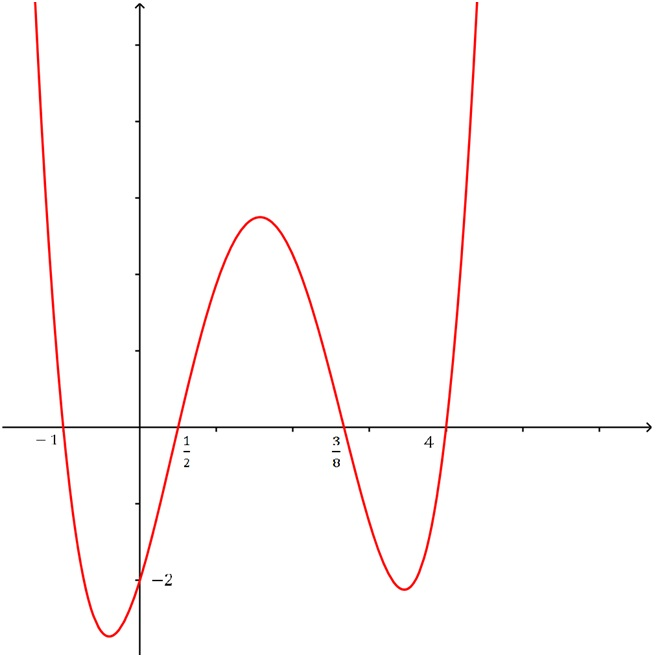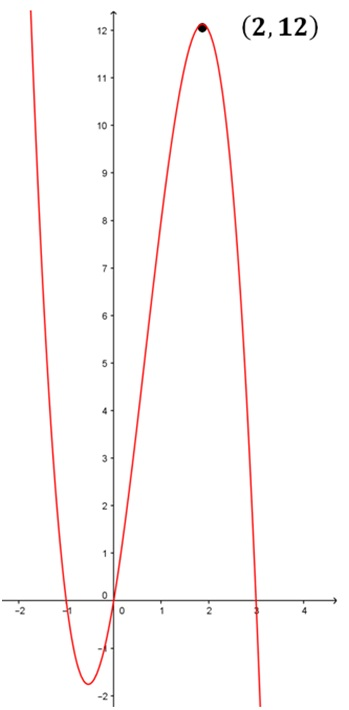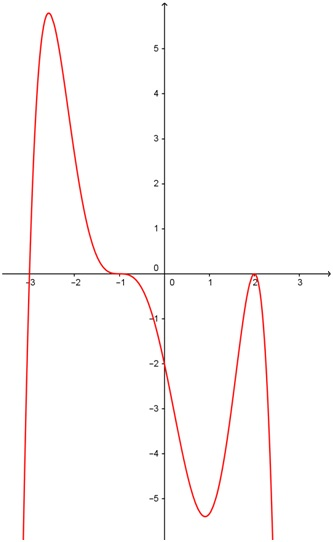# Determining the equation of a polynomial function#### All in One Place

Everything you need for better grades in university, high school and elementary.#### Learn with Ease

Made in Canada with help for all provincial curriculums, so you can study in confidence.#### Instant and Unlimited Help

0/1
##### Intros
###### Lessons
1. Steps to Finding the Equation of a Polynomial Function
0/3
##### Examples
###### Lessons
1. Determining the Equation of a Polynomial Function With Multiplicities Equal to 1
Determine the equation of the polynomial function of lowest possible degree in factored form.
1.2.2. Determining the Equation of a Polynomial Function With Higher Multiplicities
Determine the equation of the polynomial function of lowest possible degree in factored form.###### Topic Notes
We can easily find the equation of a polynomial from its graph by identifying x-intercept and the sign of the leading coefficient. That way, we can determine the factors of the polynomial and the end behavior of the function.
Steps to determining the equation of a polynomial function
1. Find the zeros, write them out in factored form.
2. Determine the multiplicity each zero by observing the behavior of the graph near the zero.
3. Determine the leading coefficient C by plugging in the coordinates of a point (other than the x-intercepts) on the graph.